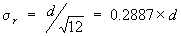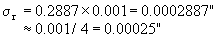# Measurement Error Due To Rounding

Dr. Wayne A. Taylor

One source of measurement error is rounding error. Rounding error is the difference between the true value and the nearest reportable value. Take the measurement of sheeting thickness as an example. If the thickness is recorded to the nearest thousandth of an inch (0.001″), then the reported values will be values like 0.013″, 0.014″, 0.015″ and 0.016″. Suppose a particular unit has a true thickness of 0.01523″. The nearest reportable value is 0.015″. The difference between these two values, 0.00023″, is the rounding error.

Rounding is not the only source of measurement error or variation. Other sources include variation due to the measurement equipment, testing materials and the checker. These other sources of measurement variation can result in readings of 0.016″ or 0.014″ even though the true value is 0.01523″ and the nearest reportable value is 0.015″.

The amount of variation that rounding causes is easily predicted without collecting any data. First, the difference between consecutive reportable values is needed. This difference will be denoted by d. In the sheeting thickness example above, the sheeting thickness is reported to the nearest thousandth of an inch resulting in values like 0.013″, 0.014″, and 0.015″. The difference between consecutive reportable values is d=0.001″.

The standard deviation of the rounding error, denoted s r, is then:As a rule of thumb, the standard deviation due to rounding is one-quarter of the difference between consecutive reportable values. For the sheeting thickness example:Based on rounding alone, the measurement error must be at least 0.00025″.

The above expression assumes that if the reported value is 0.015″, the true value is equally likely to occur anywhere over the interval from 0.0145″ to 0.0155″. The rounding standard deviation can then be determined assuming a uniform distribution over this interval.

Measurement variation can never be smaller than the rounding error. In most cases, it is larger. Let us look at several potential uses of this fact.

Example 1: A Gauge R&R study on a pressure gauge estimates the measurement standard deviation to be 0.14 lb. Pressure values are reported to the nearest half pound. This results in a standard deviation due to rounding of 0.5 / 4 = 0.125 lb. Since the measurement variation and rounding variation are almost equal, rounding is the largest source of measurement variation. Reporting the values to the nearest 0.1 lb. should reduce the measurement variation.

Example 2: A capability study on a filling machine estimates the fill volume standard deviation to be 0.01 ml. Fill volumes are reported to the nearest tenth of a ml. The standard deviation due to rounding is 0.1 / 4 = 0.025 ml. The observed variation is less than the rounding variation alone. This inconsistency indicates a problem with the way the readings are taken.

Now apply this rule to your process to find out if rounding is a major source of your variation.

Appeared in FDC Control, Food Drug & Cosmetic Division ASQ, No. 117, February 1998, p. 3